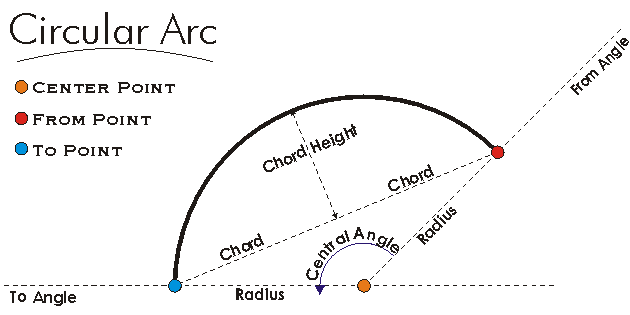ArcObjects Library Reference (Geometry)

# ICircularArc Interface

#### Product Availability

Available with ArcGIS Engine, ArcGIS Desktop, and ArcGIS Server.

#### Description

A CircularArc is an object that describes any portion of a circle.  The size of the CircularArc is determined by the length of the Radius and the Central Angle.  CircularArcs can be constructed by numerous different methods given a wide variety of input parameters.  A CircularArc differs from an EllipticArc in that every point along the CircularArc must be a fixed distance (the Radius) from the CenterPoint.

#### Members

DescriptionCenterPoint The center point.CentralAngle The included (or central) angle.ChordHeight The chord height (assigning preserves endpoints, and orientation unless chord height is < 0).Complement Changes this arc into its complement; 'from' and 'to' points are unchanged.Dimension The topological dimension of this geometry.Envelope Creates a copy of this geometry's envelope and returns it.FromAngle The angle, measured from a horizontal line through this circular arc's center point, that defines where this arc starts.FromPoint The 'from' point of the curve.GeometryType The type of this geometry.GeoNormalize Shifts longitudes, if need be, into a continuous range of 360 degrees.GeoNormalizeFromLongitude Normalizes longitudes into a continuous range containing the longitude. This method is obsolete.GetSubcurve Extracts a portion of this curve into a new curve.IsClosed Indicates if 'from' and 'to' points (of each part) are identical.IsCounterClockwise Indicates if this circular is oriented counter-clockwise from its 'from' point to its 'to' point.IsEmpty Indicates whether this geometry contains any points.IsLine Indicates if the arc has degenerated to a line (radius is infinite).IsMinor Indicates whether this circular arc is a minor arc or a major arc.IsPoint Indicates if the arc has degenerated to a point (radius is 0).Length The length of the curve.Project Projects this geometry into a new spatial reference.PutCoords Defines this arc by a center point, 'from' and 'to' points, and orientation. If the from and to points do not lie on the same circle, the arc's center point will be adjusted.PutCoordsByAngle Defines this circular arc by a center point, 'from' angle, signed central angle, and radius.PutRadiusByPoint Defines the radius of this circular arc to be the distance from the arc's center point to the input point; other properties remain unchanged.QueryCenterPoint Copies the center point of this circular arc to the input point.QueryCoords Copies the center, 'from' and 'to' points, orientation and major/minor property into the input parameters.QueryCoordsByAngle Returns the center point, 'from' angle, signed central angle, and radius.QueryEnvelope Copies this geometry's envelope properties into the specified envelope.QueryFromPoint Copies this curve's 'from' point to the input point.QueryNormal Constructs a line normal to a curve from a point at a specified distance along the curve.QueryPoint Copies to outPoint the properties of a point on the curve at a specified distance from the beginning of the curve.QueryPointAndDistance Finds the point on the curve closest to inPoint, then copies that point to outPoint; optionally calculates related items.QueryTangent Constructs a line tangent to a curve from a point at a specified distance along the curve.QueryToPoint Copies the curve's 'to' point into the input point.Radius The radius.ReverseOrientation Reverses the parameterization of the curve ('from' point becomes 'to' point, first segment becomes last segment, etc).SetEmpty Removes all points from this geometry.SnapToSpatialReference Moves points of this geometry so that they can be represented in the precision of the geometry's associated spatial reference system.SpatialReference The spatial reference associated with this geometry.ToAngle The angle, measured from a horizontal line through this circular arc's center point, that defines where this arc ends.ToPoint The 'to' point of the curve.

#### Inherited Interfaces

Interfaces Description
ICurve Provides access to properties and methods of all 1 dimensional curves (polylines, segments, boundaries of polygons, etc.).
IGeometry Provides access to members that describe properties and behavior of all geometric objects.

#### CoClasses that implement ICircularArc

CoClasses and Classes Description
CircularArc A portion of a circle that connects two points optionally has measure, height and ID attributes at each endpoint.

#### Remarks[C#]

private void OutputArcProperties(ICircularArc circularArc)
{
String report = "Center Point: (" + circularArc.CenterPoint.X + ", " +
circularArc.CenterPoint.Y + ")" + "\n" +
"From Point: (" + circularArc.FromPoint.X + ", " +
circularArc.FromPoint.Y + ")" + "\n" +
"To Point: (" + circularArc.ToPoint.X + ", " +
circularArc.ToPoint.Y + ")" + "\n" +
"From Angle: " + circularArc.FromAngle + "\n" +
"Central Angle: " + circularArc.CentralAngle + "\n" +
"To Angle: " + circularArc.ToAngle + "\n" +
"Chord Height: " + circularArc.ChordHeight + "\n" +
"IsCounterClockwise: " + circularArc.IsCounterClockwise + "\n" +
"IsMinor: " + circularArc.IsMinor + "\n" +
"IsLine: " + circularArc.IsLine + "\n" +
"IsPoint: " + circularArc.IsPoint + ")";
//IsLine and IsPoint check for degenerate cases of CircularArcs
System.Windows.Forms.MessageBox.Show(report);
}